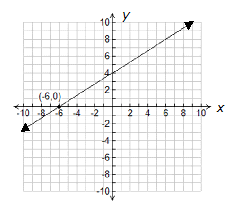﻿ Y-intercept

Y-intercept

My Definition

Key Characteristics

The following are true of the y-intercept:

• It is a point on the graph located on the y-axis.

• It is indicated by an ordered pair in the form (0, b).

• It is expressed as a single coordinate.

• It is where a graphed line intersects the y-axis.

• It is indicated algebraically by the variable, b, in the formula y = mx + b.

Example

Non-example

The x-intercept is −6 and is located at (−6,0).TEKS: 6(4)(A), 6(6)(C), 7(7)(A), 8(4)(C), 8(5)(I)